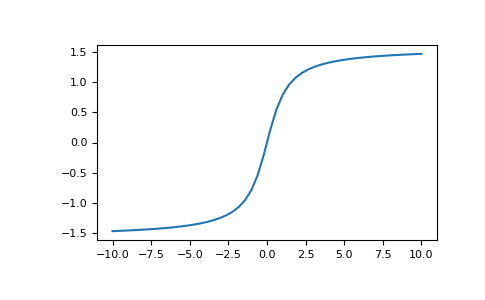# numpy.arctan¶

`numpy.``arctan`(x, /, out=None, *, where=True, casting='same_kind', order='K', dtype=None, subok=True[, signature, extobj]) = <ufunc 'arctan'>

Trigonometric inverse tangent, element-wise.

The inverse of tan, so that if `y = tan(x)` then `x = arctan(y)`.

Parameters: x : array_like out : ndarray, None, or tuple of ndarray and None, optional A location into which the result is stored. If provided, it must have a shape that the inputs broadcast to. If not provided or None, a freshly-allocated array is returned. A tuple (possible only as a keyword argument) must have length equal to the number of outputs. where : array_like, optional Values of True indicate to calculate the ufunc at that position, values of False indicate to leave the value in the output alone. **kwargs For other keyword-only arguments, see the ufunc docs. out : ndarray or scalar Out has the same shape as x. Its real part is in `[-pi/2, pi/2]` (`arctan(+/-inf)` returns `+/-pi/2`). This is a scalar if x is a scalar.

`arctan2`
The “four quadrant” arctan of the angle formed by (x, y) and the positive x-axis.
`angle`
Argument of complex values.

Notes

`arctan` is a multi-valued function: for each x there are infinitely many numbers z such that tan(z) = x. The convention is to return the angle z whose real part lies in [-pi/2, pi/2].

For real-valued input data types, `arctan` always returns real output. For each value that cannot be expressed as a real number or infinity, it yields `nan` and sets the invalid floating point error flag.

For complex-valued input, `arctan` is a complex analytic function that has [1j, infj] and [-1j, -infj] as branch cuts, and is continuous from the left on the former and from the right on the latter.

The inverse tangent is also known as atan or tan^{-1}.

References

Abramowitz, M. and Stegun, I. A., Handbook of Mathematical Functions, 10th printing, New York: Dover, 1964, pp. 79. http://www.math.sfu.ca/~cbm/aands/

Examples

We expect the arctan of 0 to be 0, and of 1 to be pi/4:

```>>> np.arctan([0, 1])
array([ 0.        ,  0.78539816])
```
```>>> np.pi/4
0.78539816339744828
```

Plot arctan:

```>>> import matplotlib.pyplot as plt
>>> x = np.linspace(-10, 10)
>>> plt.plot(x, np.arctan(x))
>>> plt.axis('tight')
>>> plt.show()
```numpy.arccos

numpy.hypot